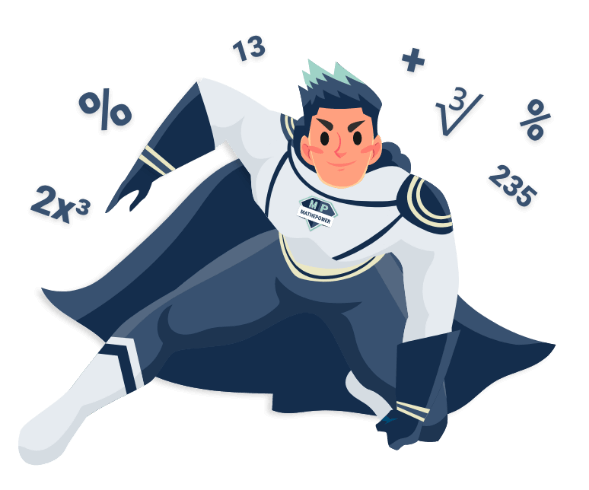# Intersection of functionsEnter one function in here. Hints: Enteras 3*x^2 ,as (x+1)/(x-2x^4) andas 3/5. Enter the other function in here. To write powers, use ^. This means, you gotta write x^2 for.

## How to find intersection points of functions?

Just equalize the functions and solve the equation you got. Then insert this solution into both functions.